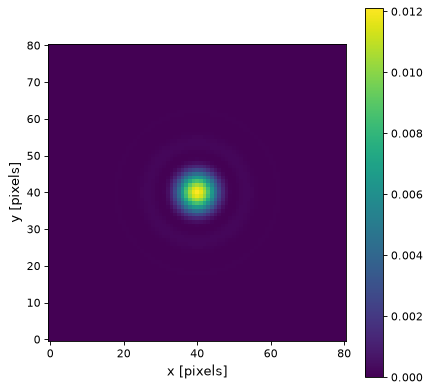# AiryDisk2DKernel¶

class `astropy.convolution.``AiryDisk2DKernel`(radius, **kwargs)[source]

2D Airy disk kernel.

This kernel models the diffraction pattern of a circular aperture.

The generated kernel is normalized so that it integrates to 1.

Parameters
radius`float`

The radius of the Airy disk kernel (radius of the first zero).

x_size`int`, optional

Size in x direction of the kernel array. Default = ⌊8*radius + 1⌋.

y_size`int`, optional

Size in y direction of the kernel array. Default = ⌊8*radius + 1⌋.

mode`str`, optional
One of the following discretization modes:
• ‘center’ (default)

Discretize model by taking the value at the center of the bin.

• ‘linear_interp’

Discretize model by performing a bilinear interpolation between the values at the corners of the bin.

• ‘oversample’

Discretize model by taking the average on an oversampled grid.

• ‘integrate’

Discretize model by integrating the model over the bin.

factornumber, optional

Factor of oversampling. Default factor = 10.

Examples

Kernel response:

```import matplotlib.pyplot as plt
from astropy.convolution import AiryDisk2DKernel
airydisk_2D_kernel = AiryDisk2DKernel(10)
plt.imshow(airydisk_2D_kernel, interpolation='none', origin='lower')
plt.xlabel('x [pixels]')
plt.ylabel('y [pixels]')
plt.colorbar()
plt.show()
```

(png, svg, pdf)